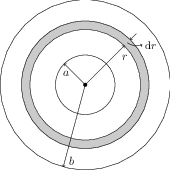# Magnetic Field Along the Axis of a Circular Coil

## Problems from IIT JEE

Problem (IIT JEE 2011):A long insulated copper wire is closely wound as a spiral of $N$ turns. The spiral has inner radius $a$ and outer radius $b$. The spiral lies in $x\text{-}y$ plane and a steady current $I$ flows through the wire. The $z$ component of magnetic field at the centre of spiral is

1. $\frac{\mu_0 N I}{2(b-a)}\ln\big(\frac{b}{a}\big)$
2. $\frac{\mu_0 N I}{2(b-a)}\ln\big(\frac{b+a}{b-a}\big)$
3. $\frac{\mu_0 N I}{2b}\ln\big(\frac{b}{a}\big)$
4. $\frac{\mu_0 N I}{2b}\ln\big(\frac{b+a}{b-a}\big)$

Solution:Consider a small circular element of thickness $\text{d}r$ at a distance $r$ from the centre of spiral (see figure). The number of turns in this element is $\frac{N}{b-a}\text{d}r$ and thus current flowing through this element is $i=\frac{N}{b-a}\text{d}rI$ (current $I$ through each turn). The magnetic field at the centre of spiral due to this element is given by \begin{alignat}{2} &\mathrm{d}B=\frac{\mu_0 i}{2r}=\frac{\mu_0 NI}{2(b-a)}\frac{\text{d}r}{r}. \nonumber \end{alignat} Integrate $\mathrm{d}B$ from $r=a$ to $r=b$ to get, \begin{alignat}{2} &B=\int_{a}^{b}\frac{\mu_0 NI}{2(b-a)}\frac{\text{d}r}{r}=\frac{\mu_0 NI}{2(b-a)}\ln\left(\frac{b}{a}\right). \nonumber \end{alignat}